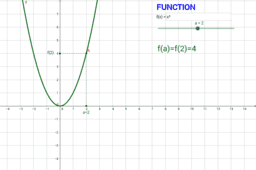# Mates y TIC - Maths and ICT

## Actividades de Matemáticas con TIC - Math Activities with ICT - - - (matesytic@gmail.com) Ricardo García Mesa

•# Archive for March 5th, 2019

## Functions I

Posted by ricardogm on 5th March 2019Hi

We are starting with functions today:

1. The basic information is in this page. You should find what a function is, what rules it has and what domain means, at least.

2. Open this Geogebra applets with a simple example of a function.Now, play with them and try to answer these questions:

a) What does f(3) mean? How much is it? How can you calculate that?

b) Are there more than one value of a with the same value of f(a)? Is that possible in a function?

3. Now we are going to create something similar. Pay attention and send me the file.

4. Read pages 40 and 41 of this book . They show you how to calculate the domain of a function.

5. With this information, solve these exercises:

domain.pdf

Solutions.You can find the same exercises with solutions in this link.

Posted in 4º ESO | No Comments »